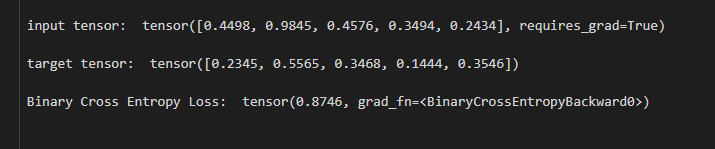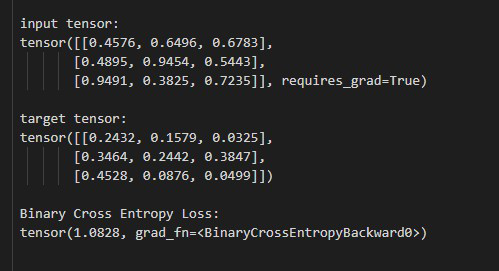GeeksforGeeks App
Open AppBrowser
Continue

# How to Measure the Binary Cross Entropy Between the Target and the Input Probabilities in PyTorch?

In this article, we are going to see how to Measure the Binary Cross Entropy between the target and the input probabilities in PyTorch using Python. We can measure this by using the BCELoss() method of torch.nn module.

## BCELoss() method

The BCELoss() method measures the Binary Cross Entropy between the target and the input probabilities by creating a criterion. This method is used for measuring the error of reconstruction, an auto-encoder is a good example of it. The input and target must be tensors of any number of dimensions and the target should be between 0 to 1. before moving further let’s see the syntax of the given method.

Syntax: torch.nn.BCELoss()

### Example 1:

In this example, we measure the Binary Cross Entropy between the target and the input probabilities of the 1D tensor.

## Python

 `# Import required library``import` `torch``import` `torch.nn as nn`` ` `# define input and target tensor``input_tens ``=` `torch.tensor(``    ``[``0.4498``, ``0.9845``, ``0.4576``, ``0.3494``, ``0.2434``],``    ``requires_grad``=``True``)``target_tens ``=` `torch.tensor([``0.2345``, ``0.5565``,``                            ``0.3468``, ``0.1444``,``                            ``0.3546``])`` ` `# display input and target tensor``print``(``'\n input tensor: '``, input_tens)``print``(``'\n target tensor: '``, target_tens)`` ` `# define criterion to measure binary ``# cross entropy``bce_loss ``=` `nn.BCELoss()`` ` `# compute the binary cross entropy``output ``=` `bce_loss(input_tens, target_tens)``output.backward()`` ` `# display result``print``(``'\n Binary Cross Entropy Loss: '``, output)`

Output:### Example 2:

In this example, we measure the Binary Cross Entropy between the target and the input probabilities of the 2D tensor.

## Python

 `# Import required library``import` `torch``import` `torch.nn as nn`` ` `# define input and target tensor``input_tens ``=` `torch.tensor([[``0.4576``, ``0.6496``, ``0.6783``],``                           ``[``0.4895``, ``0.9454``, ``0.5443``],``                           ``[``0.9491``, ``0.3825``, ``0.7235``]],``                          ``requires_grad``=``True``)`` ` `target_tens ``=` `torch.tensor([[``0.2432``, ``0.1579``, ``0.0325``],``                            ``[``0.3464``, ``0.2442``, ``0.3847``],``                            ``[``0.4528``, ``0.0876``, ``0.0499``], ])`` ` `# display input and target tensor``print``(``'\n input tensor: \n'``, input_tens)``print``(``'\n target tensor: \n'``, target_tens)`` ` `# define criterion to measure binary cross entropy``bce_loss ``=` `nn.BCELoss()`` ` `# compute the binary cross entropy``output ``=` `bce_loss(input_tens, target_tens)``output.backward()`` ` `# display result``print``(``'\n Binary Cross Entropy Loss: \n'``, output)`

Output:My Personal Notes arrow_drop_up